# Julia Fractal set in C/C++ Using Graphics

• Difficulty Level : Expert
• Last Updated : 02 Jan, 2020We provide nothing but the best curated videos and practice problems for our students. Check out the C Foundation Course and master the C language from basic to advanced level. Wait no more, start learning today!

The Julia set is associated with those points z = x + iy on the complex plane for which the series zn+1 = zn2 + c does not tend to infinity. c is a complex constant, one gets a different Julia set for each c. The initial value z0 for the series is each point in the image plane.

The well known Mandelbrot set forms a kind of index into the Julia set. A Julia set is either connected or disconnected, values of c chosen from within the Mandelbrot set are connected while those from the outside of the Mandelbrot set are disconnected. The disconnected sets are often called “dust”, they consist of individual points no matter what resolution they are viewed at.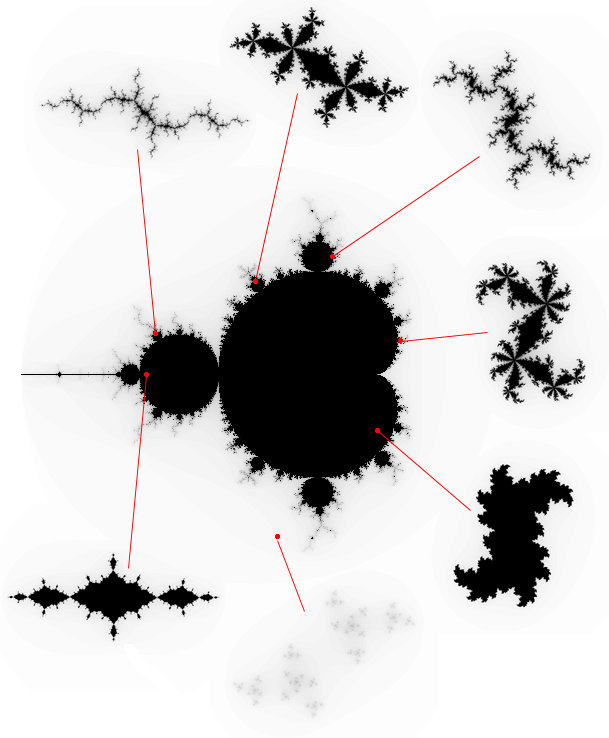### Code

 `#include ``#include ``#include ``#include `` ` `#define Y 1079``#define X 1919`` ` `// To recursively find the end value``// of the passed point till the pixel``// goes out of the bounded region``// or the maximum depth is reached.``int` `julia_point(``float` `x, ``float` `y,``                ``int` `r, ``int` `depth,``                ``int` `max,``                ``double` `_Complex c,``                ``double` `_Complex z)``{``    ``if` `(cabs(z) > r) {``        ``putpixel(x, y,``                 ``COLOR(255 - 255 * ((max - depth) * (max - depth)) % (max * max),``                       ``0, 0));``        ``depth = 0;``    ``}``    ``if` `(``sqrt``(``pow``((x - X / 2), 2)``             ``+ ``pow``((y - Y / 2), 2))``        ``> Y / 2) {``        ``putpixel(x, y, 0);``    ``}``    ``if` `(depth < max / 4) {``        ``return` `0;``    ``}``    ``julia_point(x, y, r,``                ``depth - 1, max,``                ``c, cpow(z, 2) + c);``}`` ` `// To select the points in a Julia set.``void` `juliaset(``int` `depth, ``double` `_Complex c, ``int` `r, ``int` `detail)``{``    ``for` `(``float` `x = X / 2 - Y / 2; x < X / 2 + Y / 2; x += detail) {``        ``for` `(``float` `y = 0; y < Y; y += detail) {``            ``julia_point(x, y, r,``                        ``depth, depth, c,``                        ``(2 * r * (x - X / 2) / Y)``                            ``+ (2 * r * (y - Y / 2) / Y)``                                  ``* _Complex_I);``        ``}``    ``}``}`` ` `// Driver code``int` `main()``{``    ``initwindow(X, Y);``    ``int` `depth = 100, r = 2, detail = 1;`` ` `    ``// Initial value for Julia``    ``// set taken by my personal preference.``    ``double` `_Complex c = 0.282 - 0.58 * _Complex_I;``    ``while` `(1) {`` ` `        ``cleardevice();`` ` `        ``// To formulate the display text``        ``// for the 'c' coordinate``        ``// into string format.``        ``char` `str1, str2, strtemp;``        ``if` `(``floor``(creal(c)) == -1) {``            ``strcpy``(str1, ``"-0."``);``        ``}``        ``if` `(``floor``(creal(c)) == -0) {``            ``strcpy``(str1, ``"0."``);``        ``}``        ``if` `(``floor``(cimag(c)) == -1) {``            ``strcpy``(str2, ``"-0."``);``        ``}``        ``if` `(``floor``(cimag(c)) == -0) {``            ``strcpy``(str2, ``"0."``);``        ``}``        ``itoa(``sqrt``(``pow``(creal(c), 2)) * 1000, strtemp, 10);``        ``strcat``(str1, strtemp);``        ``strcat``(str1, ``", "``);``        ``itoa(``sqrt``(``pow``(cimag(c), 2)) * 1000, strtemp, 10);``        ``strcat``(str2, strtemp);``        ``strcat``(str1, str2);``        ``outtextxy(X * 0.8, Y * 0.8, str1);`` ` `        ``// To call the julia-set for the selected value of 'c'.``        ``juliaset(depth, c, r, detail);``        ``outtextxy(X / 3, Y * 0.9,``                  ``"Press '1' to Exit, Space to"``                  ``" select a point or any "``                  ``"other key to continue"``);``        ``char` `key = getch();`` ` `        ``if` `(key == ``'\n'``) {``            ``break``;``        ``}`` ` `        ``// To select the value of 'c'``        ``// using the position of the mouse and then``        ``// normalizing it between a value of -1-1i and 1+1i.``        ``while` `(key == ``' '``) {`` ` `            ``c = 2 * (``double``)(mousex() - X / 2) / X + 2 * (mousey() - Y / 2) * _Complex_I / Y;`` ` `            ``if` `(``floor``(creal(c)) == -1) {``                ``strcpy``(str1, ``"-0."``);``            ``}`` ` `            ``if` `(``floor``(creal(c)) == -0) {``                ``strcpy``(str1, ``"0."``);``            ``}`` ` `            ``if` `(``floor``(cimag(c)) == -1) {``                ``strcpy``(str2, ``"-0."``);``            ``}`` ` `            ``if` `(``floor``(cimag(c)) == -0) {``                ``strcpy``(str2, ``"0."``);``            ``}`` ` `            ``itoa(``sqrt``(``pow``(creal(c), 2))``                     ``* 1000,``                 ``strtemp, 10);``            ``strcat``(str1, strtemp);``            ``strcat``(str1, ``", "``);``            ``itoa(``sqrt``(``pow``(cimag(c), 2))``                     ``* 1000,``                 ``strtemp, 10);``            ``strcat``(str2, strtemp);``            ``strcat``(str1, str2);``            ``outtextxy(X * 0.8, Y * 0.8, str1);``            ``if` `(kbhit()) {``                ``key = getch();``            ``}``        ``}``    ``}``    ``closegraph();``    ``return` `0;``}`

### Output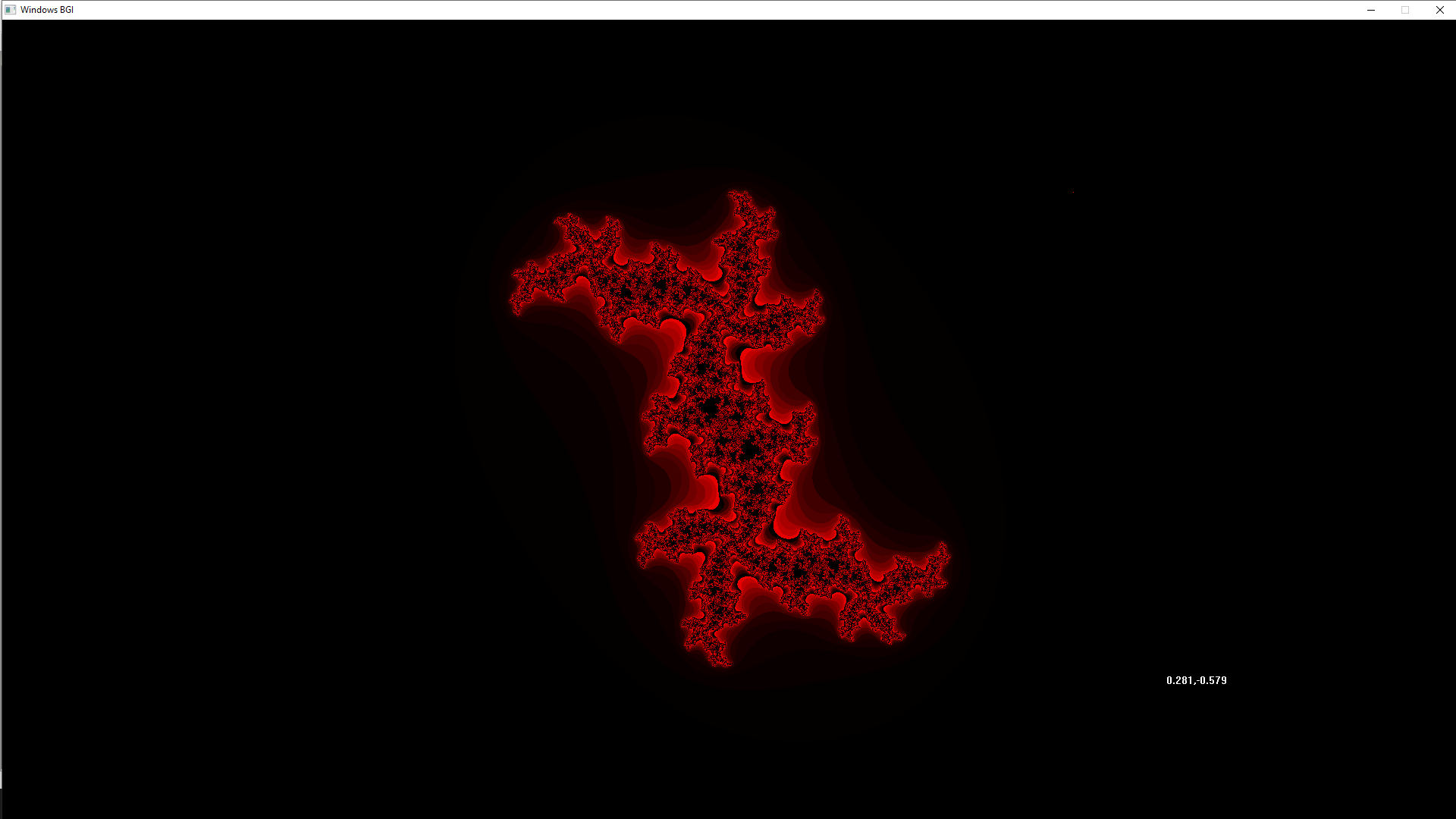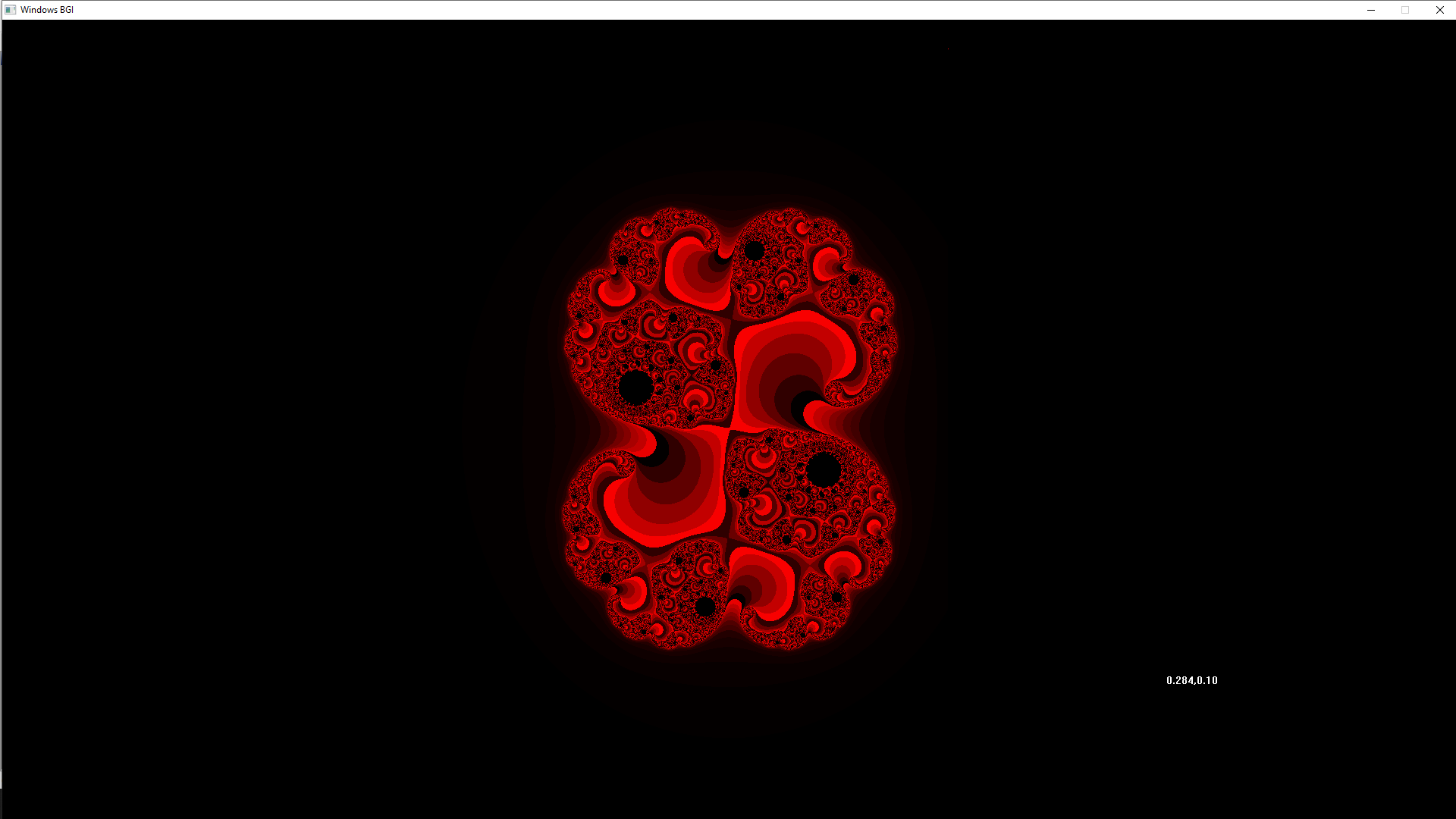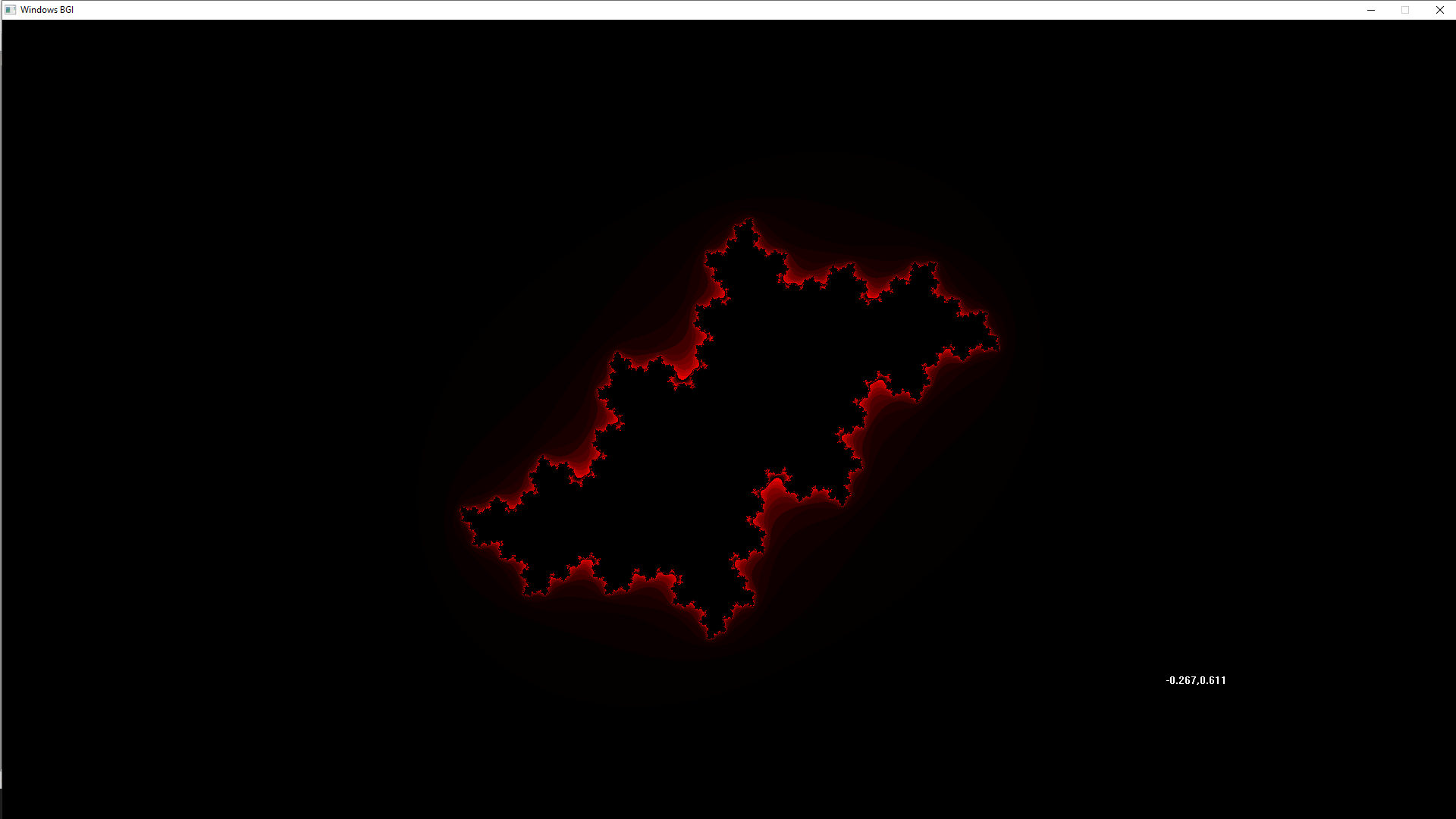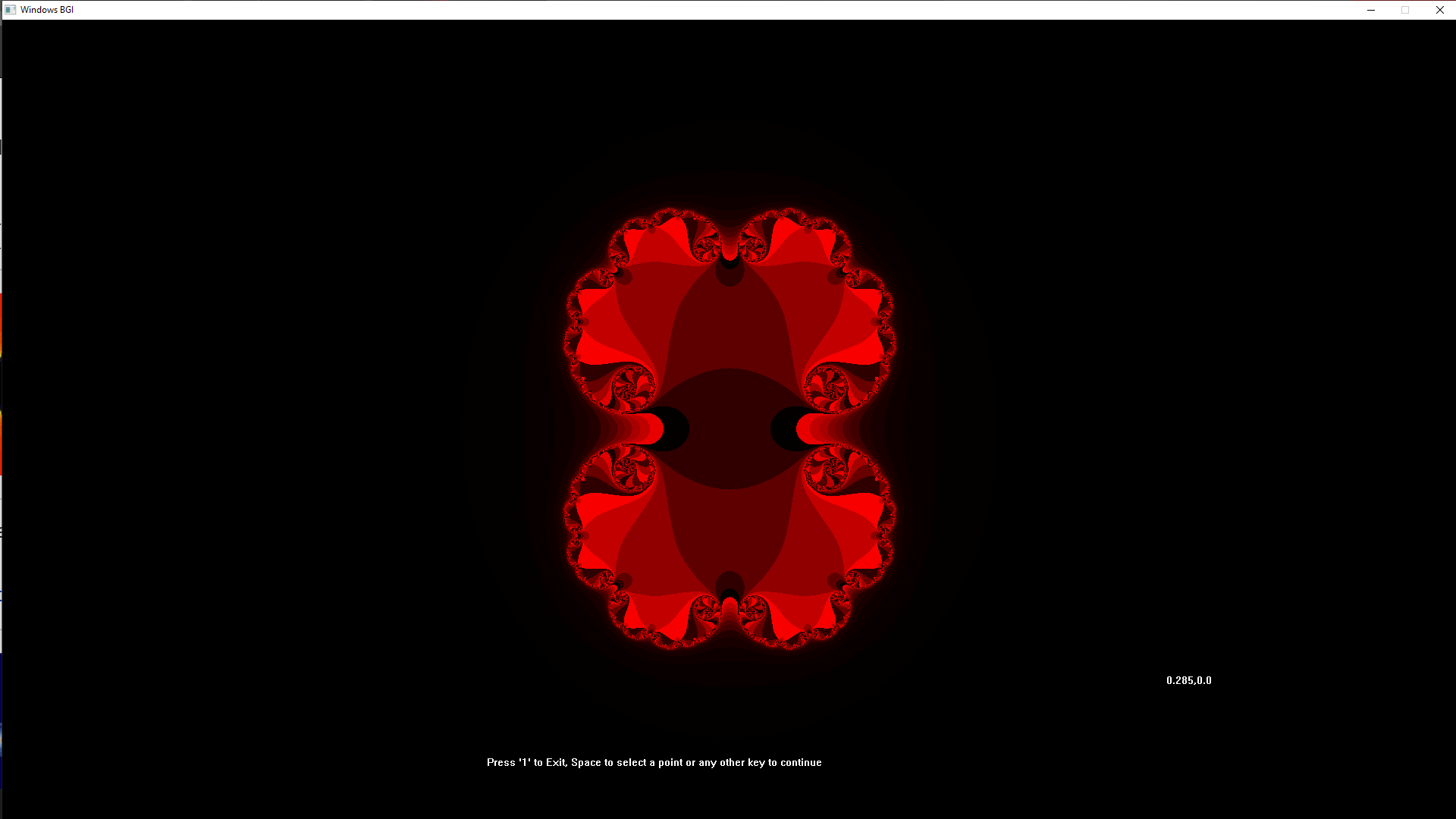My Personal Notes arrow_drop_up